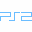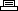Site SearchSimpson's SkateboardingPrinter Friendly Version

```All boards:
Hold L1 + L2 + R1 + R2 and press X, Triangle,
Circle, Square at the character selection screen.

Level select:
Hold L1 + L2 + R1 + R2 and press Triangle, X,
Square, Circle at the character selection screen.

Get \$99.00:
Hold L1 + L2 + R1 + R2 and press Triangle, X,
Circle, Square at the character selection screen.

All skaters:
Hold L1 + L2 + R1 + R2 and press Circle,
Triangle, X, Square at the character selection screen.

Fuzzy skaters:
Hold L1 + L2 + R1 + R2 and press X, Triangle,
Square, Circle at the character selection screen.

Hold L1 + L2 + R1 + R2 and press Circle, X,
Triangle, Square at the character selection screen.

Underwear Homer:
Hold L1 + L2 + R1 + R2 and press Triangle, Circle,
X, Square at the character selection screen.

Hold L1 + L2 + R1 + R2 and press X, Square, Circle,
Triangle at the character selection screen.

Gangsta Bart:
Hold L1 + L2 + R1 + R2 and press Circle, X, Square,
Triangle at the character selection screen.

Demon Marge:
Hold L1 + L2 + R1 + R2 and press X, Square, Triangle,
Circle at the character selection screen.

Hold L1 + L2 + R1 + R2 and press Square, Triangle, X,
Circle at the character selection screen.

Gangsta Lisa:
Hold L1 + L2 + R1 + R2 and press Square, Triangle,
Circle, X at the character selection screen.

Hold L1 + L2 + R1 + R2 and press Triangle, Square,
Circle, X at the character selection screen.

Ballerina Nelson:
Hold L1 + L2 + R1 + R2 and press Triangle, Square, X,
Circle at the character selection screen.

Sunglasses Otto:
Hold L1 + L2 + R1 + R2 and press Square, X, Circle,
Triangle at the character selection screen.

Hold L1 + L2 + R1 + R2 and press Square, X, Triangle,
Circle at the character selection screen.

Groovy Frink:
Hold L1 + L2 + R1 + R2 and press X, Circle, Triangle,
Square at the character selection screen.

Hold L1 + L2 + R1 + R2 and press Circle, Triangle,
Square, X at the character selection screen.

Hold L1 + L2 + R1 + R2 and press X, Circle, Square,
Triangle at the character selection screen.

Man eater Wiggum:
Hold L1 + L2 + R1 + R2 and press Triangle, Circle,
Square, X at the character selection screen.

Unlocking cheats:
Successfully complete the game as any character or collecting
all items in Skatefest with Chief Wiggum to unlock a cheat.

Bonus skaters:
Finish in first place on two different Timed Trick Contest
stages to unlock an additional skater.
```